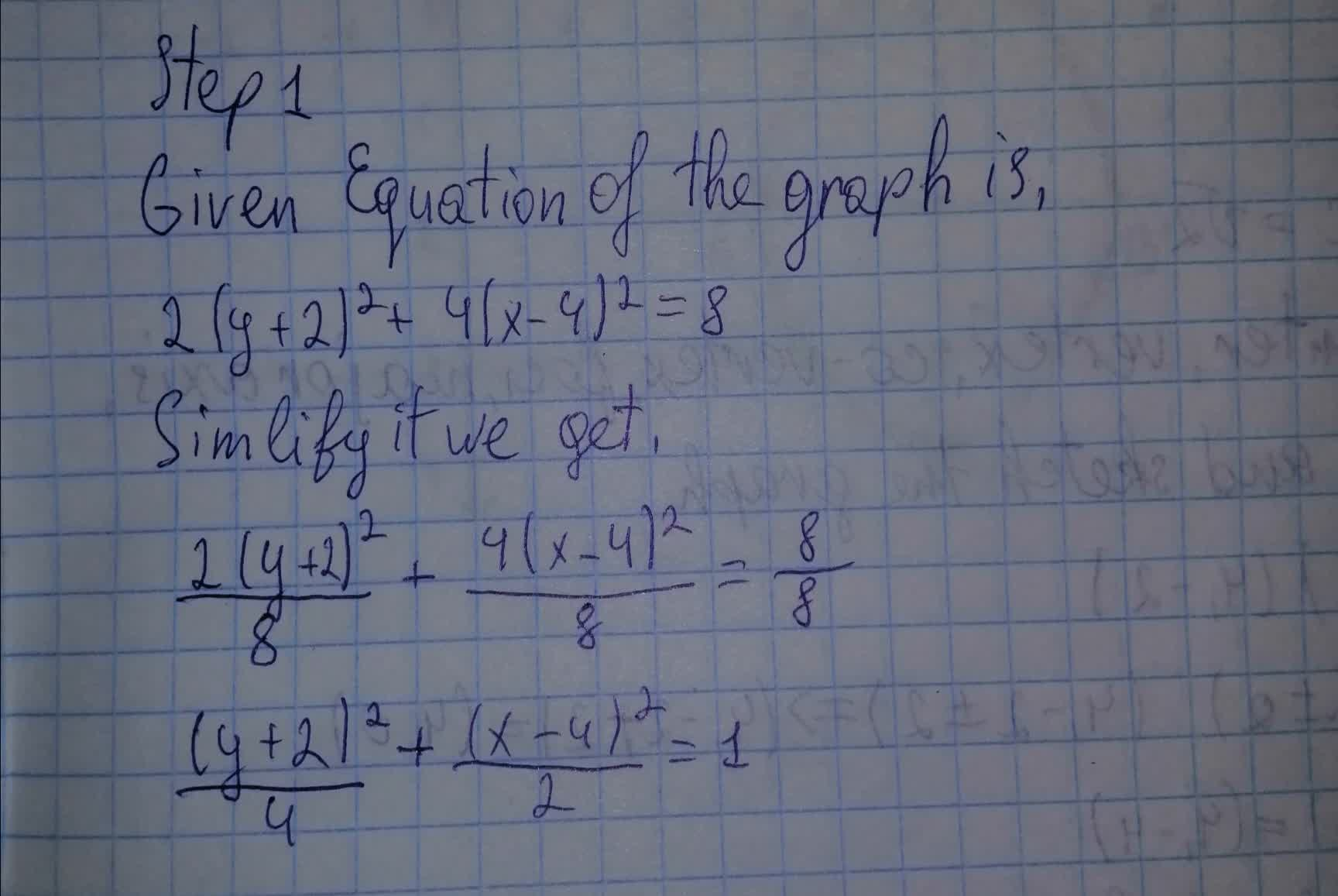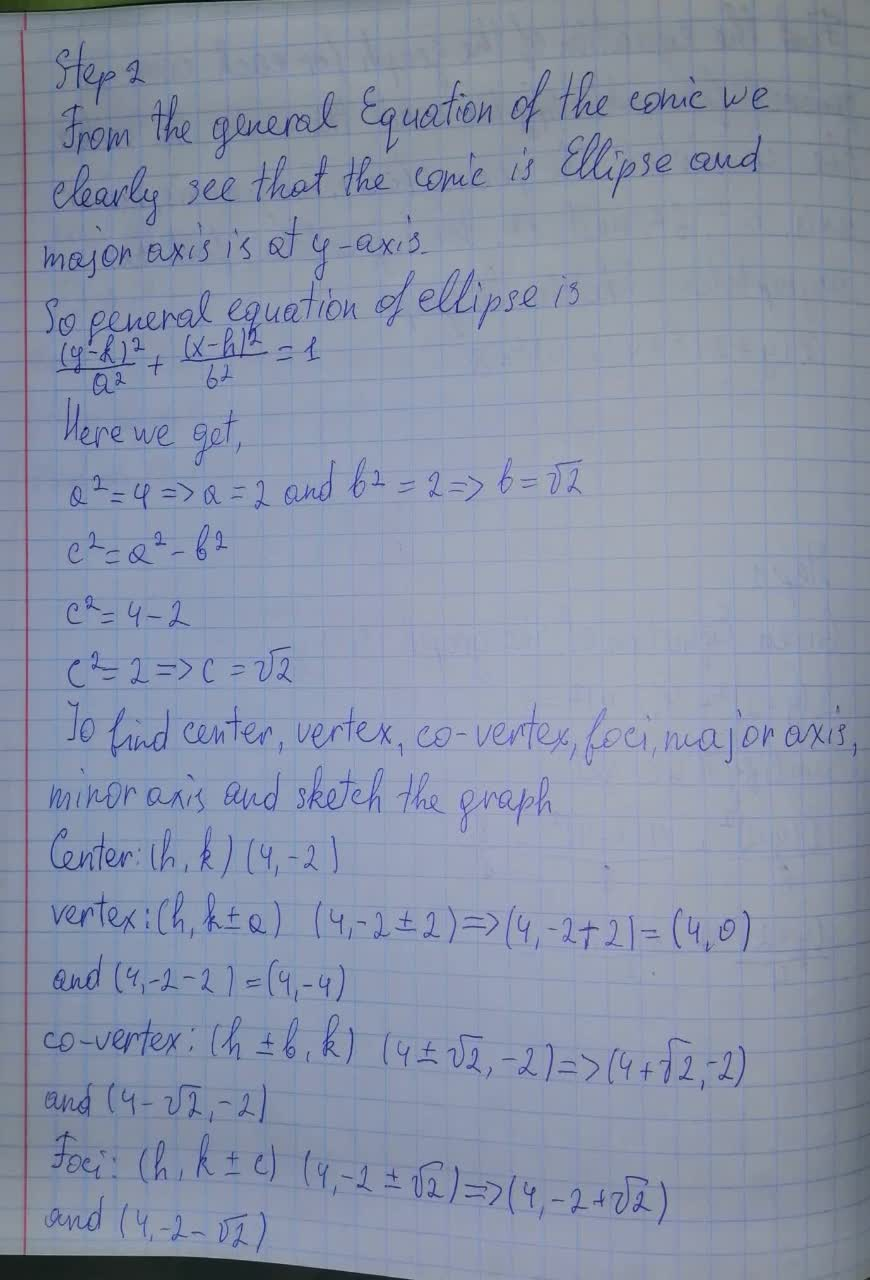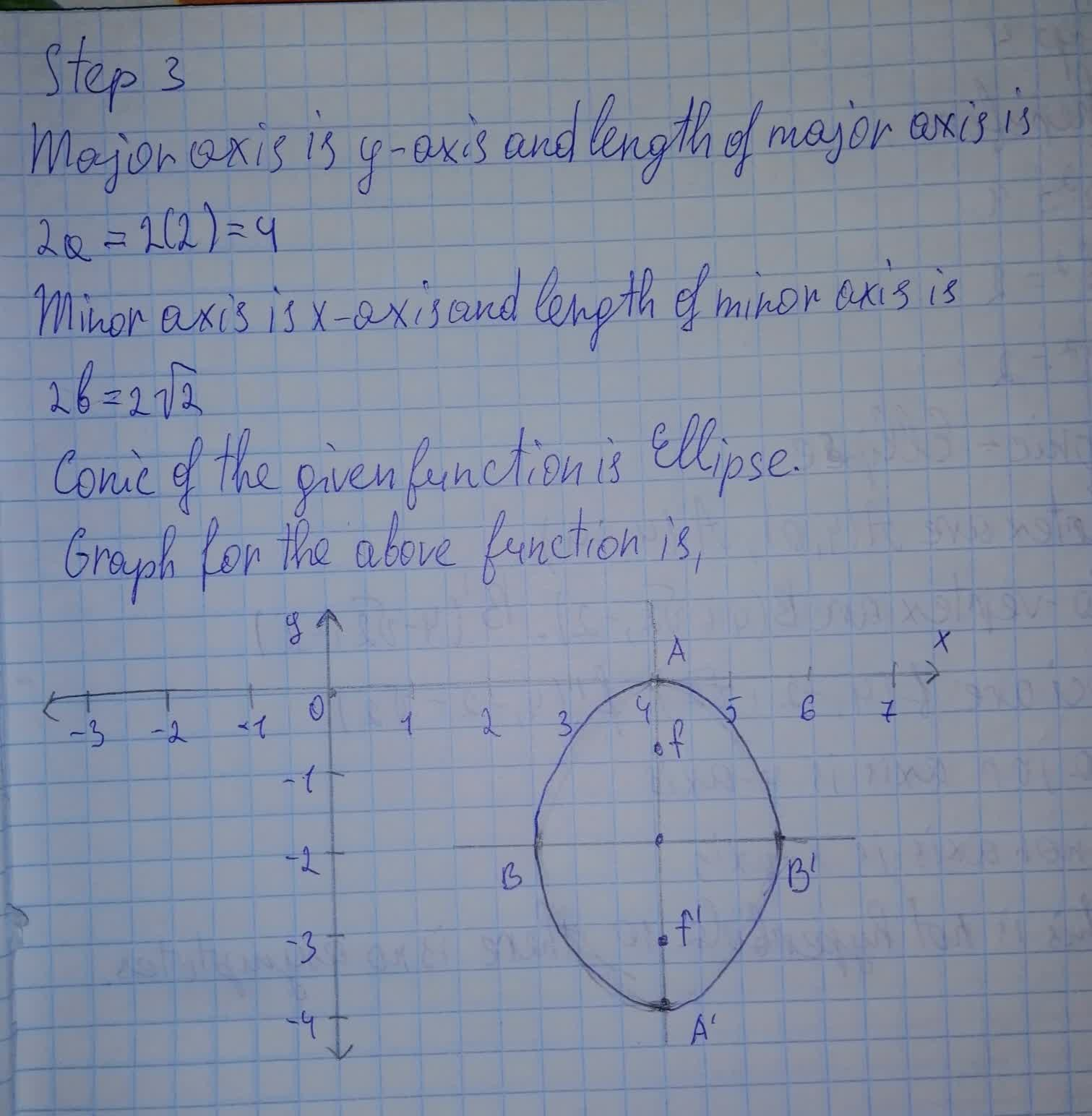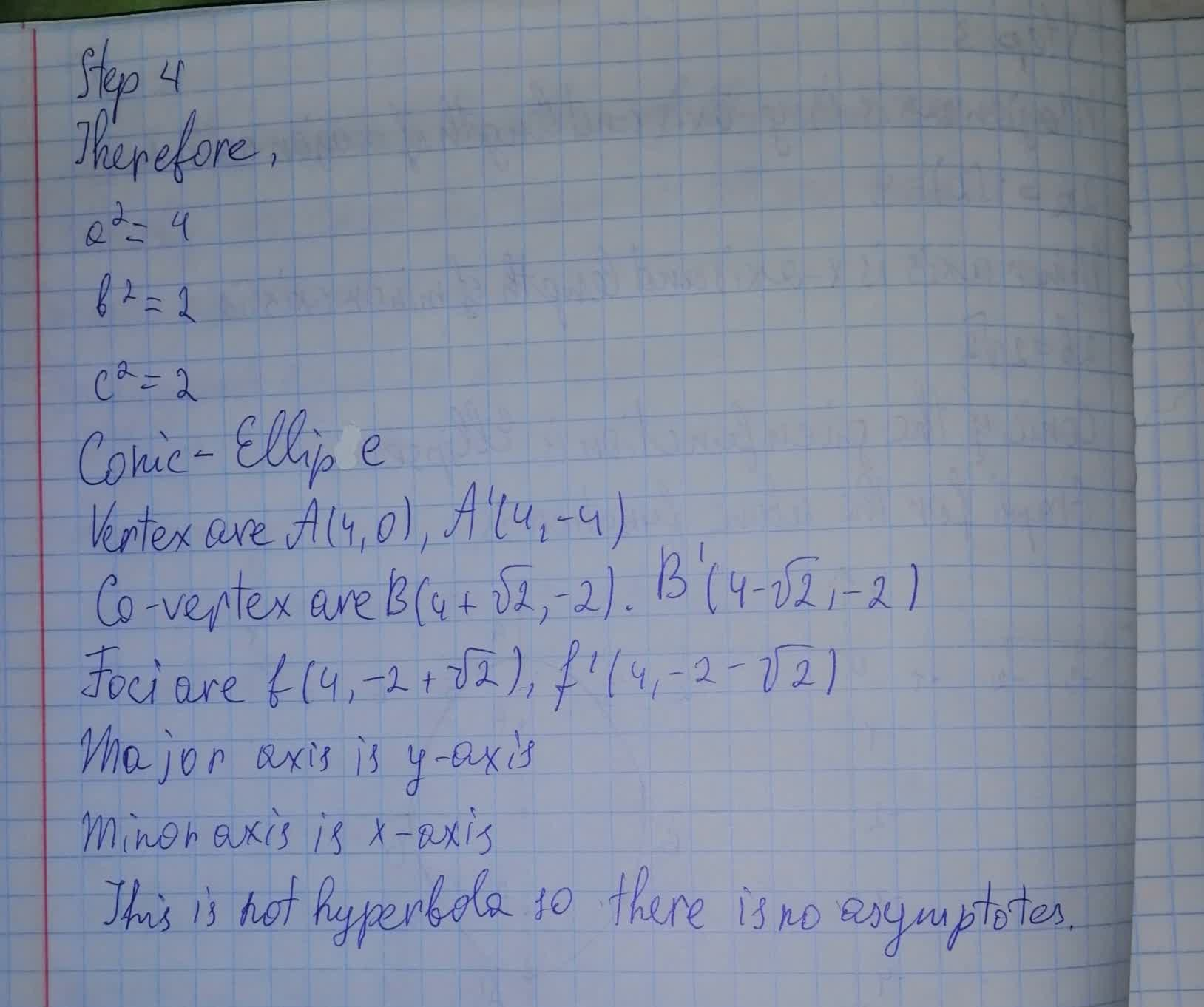# Find the equation of the graph for each conic in general form.Sketch the graph 2(y+2)^2+4(x-4)^2=8ossidianaZ 2021-08-10 Answered
Find the equation of the graph for each conic in general form. Identify the conic, the center, the vertex, the co-vertex, the focus (foci), major axis, minor axis, $$\displaystyle{a}^{{2}}$$, $$\displaystyle{b}^{{2}}$$, and $$\displaystyle{c}^{{2}}$$. For hyperbola,find the asymtotes.Sketch the graph
$$\displaystyle{2}{\left({y}+{2}\right)}^{{2}}+{4}{\left({x}-{4}\right)}^{{2}}={8}$$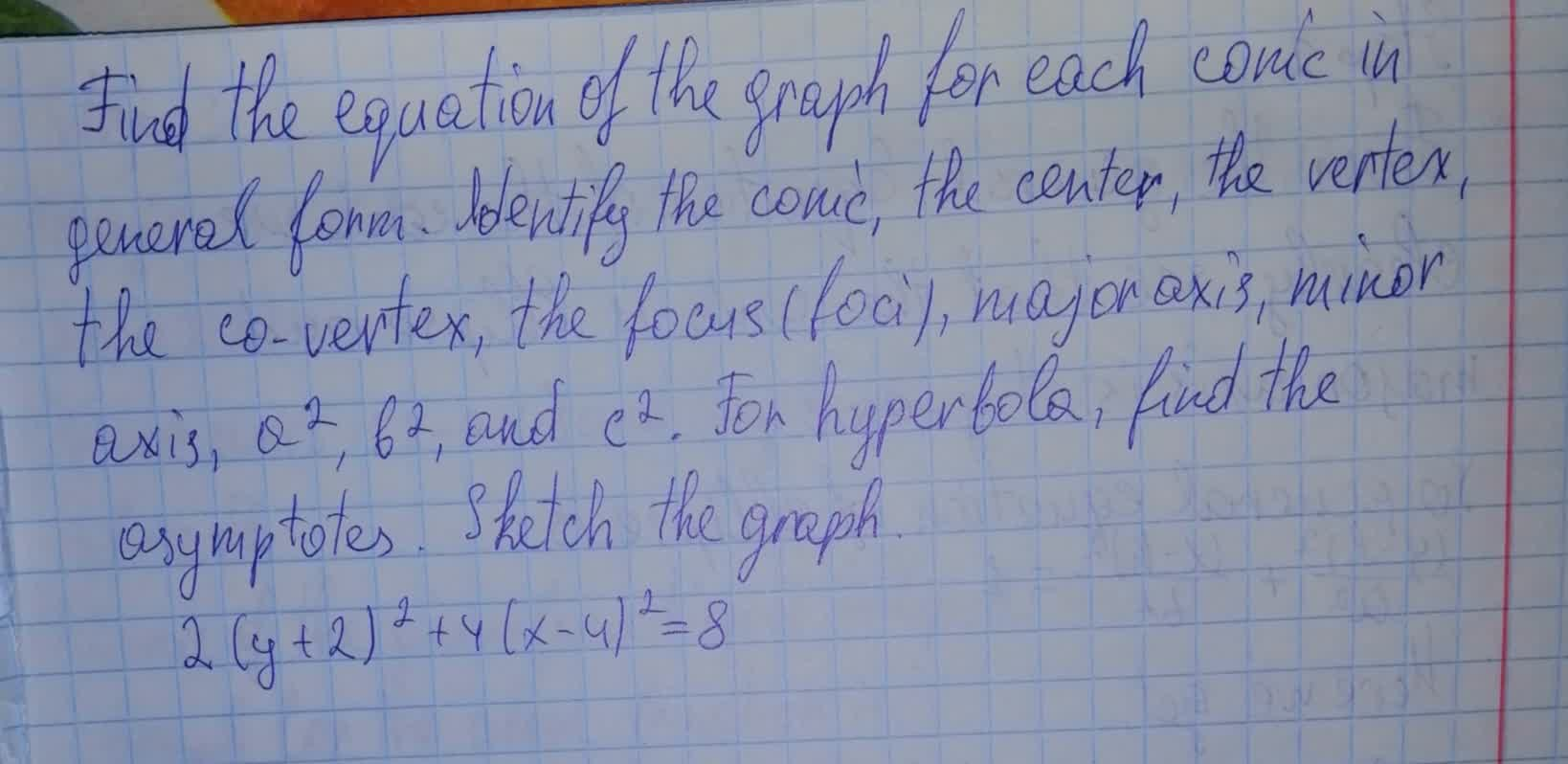• Questions are typically answered in as fast as 30 minutes

### Plainmath recommends

• Get a detailed answer even on the hardest topics.
• Ask an expert for a step-by-step guidance to learn to do it yourself.oppturf